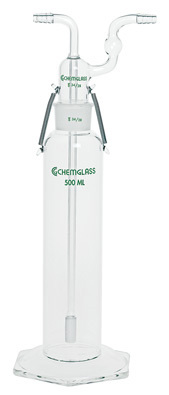MSDSTopics Free Sites FAQ's Regulations Glossary Software Suppliers Books Forum Poll Fun stuff Quiz Store Understand your MSDS with the MS-Demystifier Search ALL our MSDS info

Volume Unit ConversionsSafety Emporium carries all kinds of eye/face washes, safety showers, drench hoses and more.

### Definition

The volume of an object describes the amount of three-dimensional space that it physically occupies.

Volumes appear on Safety Data Sheets in many contexts, such as the density of the material or the concentrations that pose a health or physical hazard. Some sheets may use either traditional English measurements (ounces, gallons, etc.) or metric ones (milliters, liters etc.). Not all sheets use both conventions, so here is a handy conversion calculator for some common measures of volume. The definitions of each unit are in the next section.

 Volume Conversion Calculator(Enter number on the left side; answer appears on the right side) This many: ouncespintsquartsgallonscubic inchescubic feetcubic yardsmicrolitersmilliliters (cc) decilitersliterscubic meters Equals ouncespintsquartsgallonscubic inchescubic feetcubic yardsmicrolitersmilliliters (cc) decilitersliterscubic meters

There are a variety of U.S. Customary, British Imperial and metric (SI) units for volume. Why is metric the best choice? Consider:

• The U.S. has two definitions of the pint and quart called the dry and wet measures. Each has a different size, something most Americans probably don't even realize.
• The U.S. system has an ounce which refers to a fluid volume, but also an ounce that refers to mass; these are not interchangeable. In general, an ounce of liquid does not weigh an ounce!
• The British Imperial system of liquid and dry measure uses only one ounce, pint and quart, but these differ from any of the U.S. measures as does the British Imperial gallon, peck and bushel.

This document uses only U.S. Customary liquid measures and metric units that are relevant to SDSs.

Many of the items you will find on an Safety Data Sheet come in both English (U.S. Customary System) and metric (International System or SI or cgs) units. The metric system has been adopted by almost every country except the United States. Even in the U.S., scientists and technical people use the metric system because of its ease of use.

Ounce
(oz, U.S.)
One fluid ounce = 1/8 of a half-pint = 1/16 of a pint = 1/32 of a quart = 1/128 of a gallon. One fluid ounce = 29.5735 milliliters.
Pint
(pt, U.S.)
There are two half-pints = 16 ounces in a pint. There are 2 pints in a quart and 8 pints in a gallon. One pint = 473.176 milliliters.
Quart
(qt, U.S.)
There are 4 half-pints = 2 pints = 32 ounces in a quart. There are 4 quarts in a gallon. 1 quart = 0.94635 liters = 946.35 milliliters.
Gallon
(gal, U.S.)
There are 231 cubic inches = 128 ounces = 8 pints = 4 quarts in a gallon. 1 gallon = 3.785 liters.
Cubic inches
(in3, cub. in., U.S.)
This is the volume occupied by a cube one inch on a side. 1 cubic inch = 16.387 cubic centimeters (cc and mL), There are 1728 cubic inches in one cubic foot and 231 cubic inches in one gallon.
Cubic feet
(ft3, cub. ft., U.S.)
This is the volume occupied by a cube one foot on a side. 1 cubic foot = 1728 cubic inches = 28,316.8 cubic centimeters (cc, cm3, mL)
Cubic yard
(yd3, cub. yd., U.S.)
This is the volume occupied by a cube one yard on a side. 1 cubic yard = 27 cubic feet = 0.7646 cubic meters.
Microliter
(μL, metric)
The prefix "micro" means 1/1,000,000, so 1 microliter = 0.001 milliliters. Alternatively, there are 1,000 microliters in milliliter. One microliter = 0.0000338 fluid ounces.
Milliliter
(mL, cc, cm3, metric)
The prefix "milli" means 1/1000, so 1 milliliter = 0.001 liters. Alternatively, there are 1000 milliliters in a liter. One milliliter = 0.0338 fluid ounces. Also known as a cubic centimeter (see distance units) because a cube 1 cm on each side has a volume of 1 ml. Because water has a density of 1.0, one ml of water weighs 1 gram.
Deciliter
(dl, metric)
The prefix "deci" means 1/10, so 1 deciliter = 0.10 liters. (Try not to confuse this with the decaliter which is equal to 10 liters). There are 10 deciliters in a liter.
Liter
(l, metric)
1 liter is the volume of a cube that is 10 cm (1 decimeter) on each side (see distance units). There are 10 deciliters = 1,000 milliliters = 1,000 cubic centimeters = 1.057 quarts = 33.814 ounces in a liter. Because water has a density of 1.0, one liter of water weighs 1,000 grams = 1 kilogram.
Cubic Meter
(m3, m3, metric)
This is the volume of a cube 1 meter (see distance units) on each side. One cubic meter = 1000 liters. Because water has a density of 1.0, one cubic meter of water weighs 1 metric ton = 1,000 kilograms = 1,000,000 grams (see mass units).Safety Emporium offers an extensive line of glassware for your volume needs.

### SDS Relevance

Be very careful to note the units when reading numbers on an Safety Data Sheet. If you ever perform a calculation of any sort, always remember to write the units next to each number in your calculation and make sure that they cancel properly. See our distance units entry for examples of how to properly cancel units.